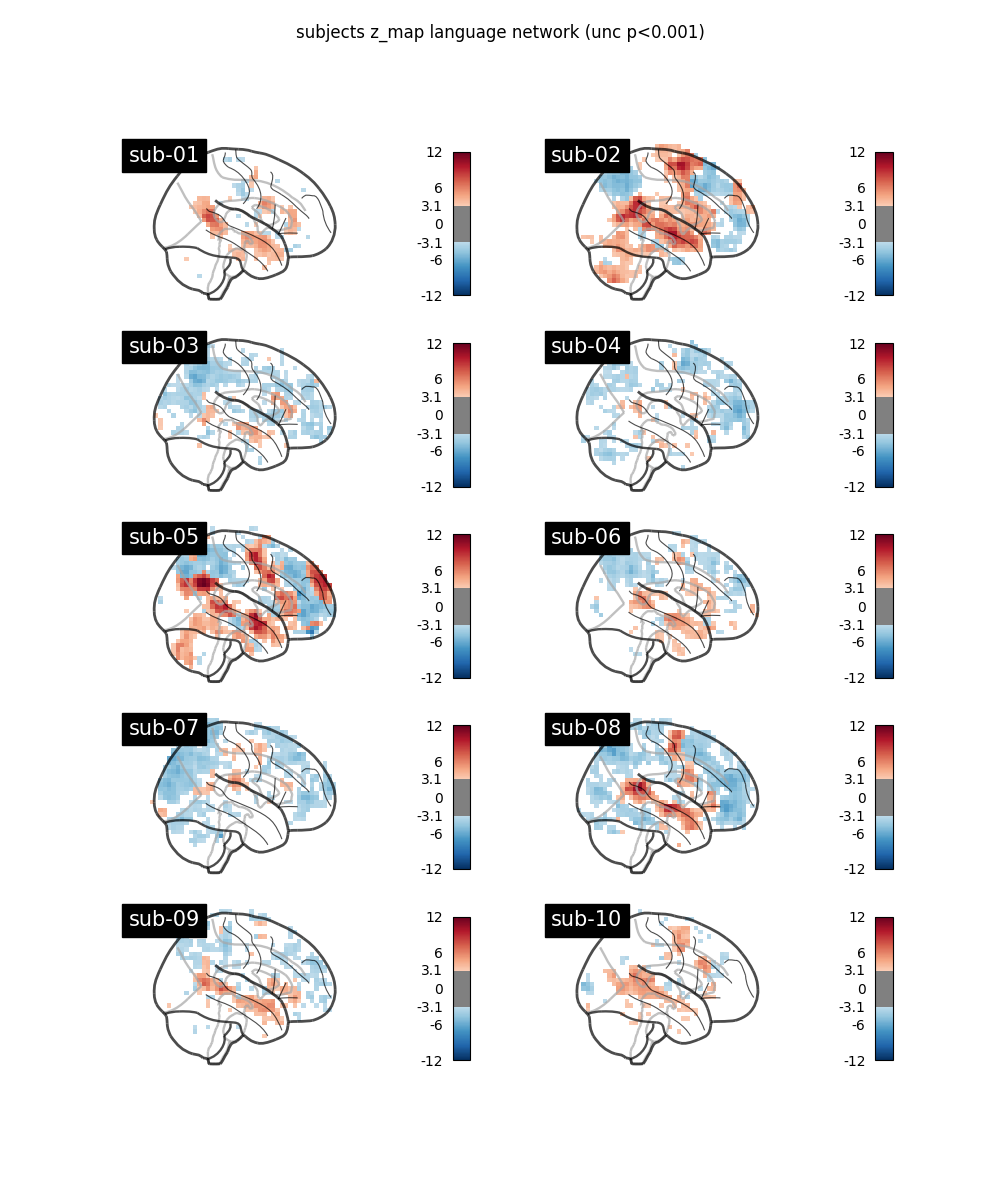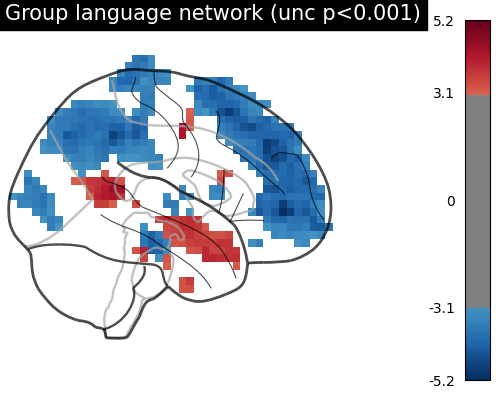# BIDS dataset first and second level analysis#

Full step-by-step example of fitting a GLM to perform a first and second level analysis in a BIDS dataset and visualizing the results. Details about the BIDS standard can be consulted at http://bids.neuroimaging.io/.

More specifically:

1. Download an fMRI BIDS dataset with two language conditions to contrast.

2. Extract first level model objects automatically from the BIDS dataset.

3. Fit a second level model on the fitted first level models. Notice that in this case the preprocessed bold images were already normalized to the same MNI space.

## Fetch example BIDS dataset#

We download a simplified BIDS dataset made available for illustrative purposes. It contains only the necessary information to run a statistical analysis using Nilearn. The raw data subject folders only contain bold.json and events.tsv files, while the derivatives folder includes the preprocessed files preproc.nii and the confounds.tsv files.

```from nilearn.datasets import fetch_language_localizer_demo_dataset

data_dir, _ = fetch_language_localizer_demo_dataset()
```

Here is the location of the dataset on disk.

```print(data_dir)
```
```/home/runner/work/nilearn/nilearn/nilearn_data/fMRI-language-localizer-demo-dataset
```

## Obtain automatically FirstLevelModel objects and fit arguments#

From the dataset directory we automatically obtain the FirstLevelModel objects with their subject_id filled from the BIDS dataset. Moreover, we obtain for each model a dictionary with run_imgs, events and confounder regressors since in this case a confounds.tsv file is available in the BIDS dataset. To get the first level models we only have to specify the dataset directory and the task_label as specified in the file names.

```from nilearn.glm.first_level import first_level_from_bids

(
models,
models_run_imgs,
models_events,
models_confounds,
) = first_level_from_bids(
)
```
```/usr/share/miniconda3/envs/testenv/lib/python3.9/site-packages/nilearn/interfaces/bids/query.py:49: UserWarning:

```

## Quick sanity check on fit arguments#

Additional checks or information extraction from pre-processed data can be made here.

We just expect one run_img per subject.

```import os

print([os.path.basename(run) for run in models_run_imgs])
```
```['sub-07_task-languagelocalizer_desc-preproc_bold.nii.gz']
```

The only confounds stored are regressors obtained from motion correction. As we can verify from the column headers of the confounds table corresponding to the only run_img present.

```print(models_confounds.columns)
```
```Index(['RotX', 'RotY', 'RotZ', 'X', 'Y', 'Z'], dtype='object')
```

During this acquisition the subject read blocks of sentences and consonant strings. So these are our only two conditions in events. We verify there are 12 blocks for each condition.

```print(models_events["trial_type"].value_counts())
```
```trial_type
language    12
string      12
Name: count, dtype: int64
```

## First level model estimation#

Now we simply fit each first level model and plot for each subject the contrast that reveals the language network (language - string). Notice that we can define a contrast using the names of the conditions specified in the events dataframe. Sum, subtraction and scalar multiplication are allowed.

Set the threshold as the z-variate with an uncorrected p-value of 0.001.

```from scipy.stats import norm

p001_unc = norm.isf(0.001)
```

Prepare figure for concurrent plot of individual maps.

```import matplotlib.pyplot as plt

from nilearn import plotting

fig, axes = plt.subplots(nrows=2, ncols=5, figsize=(8, 4.5))
model_and_args = zip(models, models_run_imgs, models_events, models_confounds)
for midx, (model, imgs, events, confounds) in enumerate(model_and_args):
# fit the GLM
model.fit(imgs, events, confounds)
# compute the contrast of interest
zmap = model.compute_contrast("language-string")
plotting.plot_glass_brain(
zmap,
colorbar=False,
threshold=p001_unc,
title=f"sub-{model.subject_label}",
axes=axes[int(midx / 5), int(midx % 5)],
plot_abs=False,
display_mode="x",
)
fig.suptitle("subjects z_map language network (unc p<0.001)")
plotting.show()
```## Second level model estimation#

We just have to provide the list of fitted FirstLevelModel objects to the SecondLevelModel object for estimation. We can do this because all subjects share a similar design matrix (same variables reflected in column names).

```from nilearn.glm.second_level import SecondLevelModel

second_level_input = models
```

Note that we apply a smoothing of 8mm.

```second_level_model = SecondLevelModel(smoothing_fwhm=8.0)
second_level_model = second_level_model.fit(second_level_input)
```

Computing contrasts at the second level is as simple as at the first level. Since we are not providing confounders we are performing a one-sample test at the second level with the images determined by the specified first level contrast.

```zmap = second_level_model.compute_contrast(
first_level_contrast="language-string"
)
```

The group level contrast reveals a left lateralized fronto-temporal language network.

```plotting.plot_glass_brain(
zmap,
colorbar=True,
threshold=p001_unc,
title="Group language network (unc p<0.001)",
plot_abs=False,
display_mode="x",
figure=plt.figure(figsize=(5, 4)),
)
plotting.show()
```Total running time of the script: (0 minutes 43.488 seconds)

Estimated memory usage: 116 MB

Gallery generated by Sphinx-Gallery